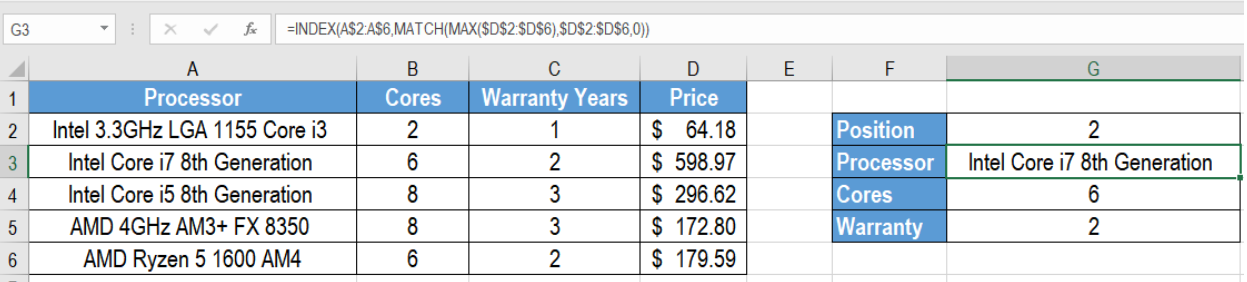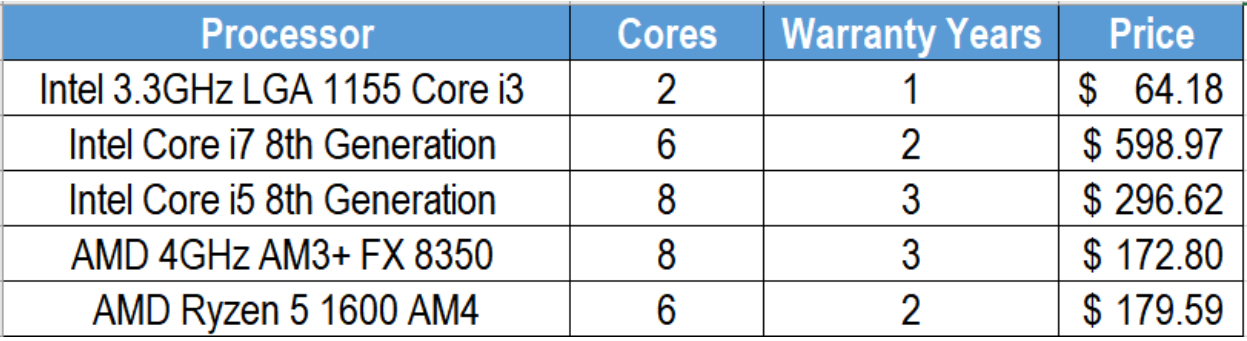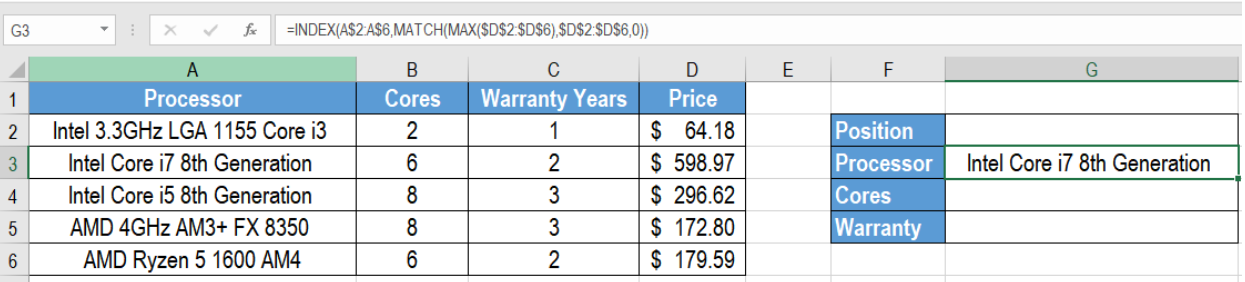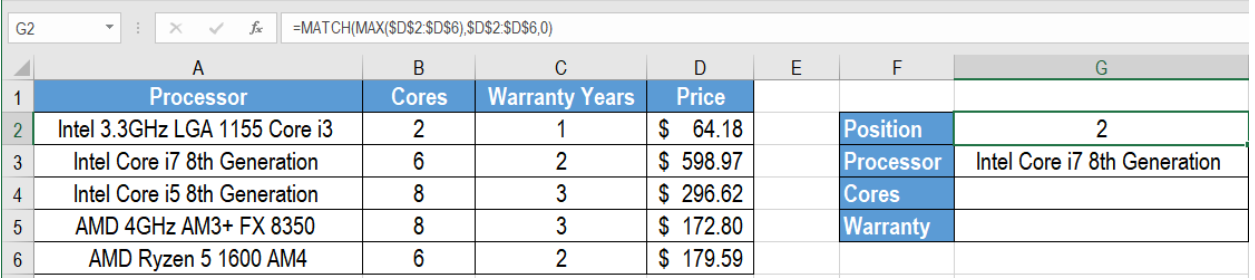Get instant live expert help with Excel or Google Sheets“My Excelchat expert helped me in less than 20 minutes, saving me what would have been 5 hours of work!”

#### Post your problem and you’ll get Expert help in seconds.

Your message must be at least 40 characters
Our professional Expert are available now. Your privacy is guaranteed.

# How to Get Information Corresponding to Max Value in Excel

Excel has some very efficient ways to find the max value in a list or range. We can also find the corresponding values of the greatest value easily. We can combine the INDEX, MATCH and MAX functions to extract the corresponding values. In this tutorial, we will learn how to get information corresponding to max value in Excel.Figure 1. Example of Getting Information Corresponding to Max Value

## Generic Formula

`=INDEX(Range1,MATCH(MAX(Range2),Range2,0)`

## How the Formula Works

Firstly, the function MAX gets the max value from Range2. We provide this value to the MATCH function as the first argument which is the lookup_value. Here, The lookup_array is also Range2. The match_type is set to 0 which represents an exact match.

The MATCH function returns the relative position of the maximum value in Range2. We supply this value to the INDEX function as the second argument. This is the row_number. The range is Range1, which is the lookup_array. Thus, the INDEX function returns the value in the relative position in Range1. This is based on the max value in Range2.

## Setting Up Data

The following example uses a microprocessor information data set. The processor names are in column A. Column B, C and D contains the price, cores and warranty years.Figure 2. The Sample Data Set

To get the corresponding information to the max value:

• We need to select cell G3.
• Assign the formula `=INDEX(A\$2:A\$6,MATCH(MAX(\$D\$2:\$D\$6),\$D\$2:\$D\$6,0)) `to cell G3.
• Press Enter.Figure 3. Assigning the Formula to Get the Corresponding Values to the Max Value

• Drag the formula using the fill handle in the bottom right to copy the formula to the cells below. We have to change the lookup_array reference to the respective columns to get the appropriate result.
• To get the position in cell G2, we need to assign the formula `=MATCH(MAX(\$D\$2:\$D\$6),\$D\$2:\$D\$6,0) `to cell G2.Figure 4. Getting the Position of the Max Value

This will find the max value which is \$598.97 and display the corresponding information in column G.

## Notes

• If there are duplicate values, this formula returns the first match.
• If the values in Range2 are not valid numbers, this formula returns an #VALUE error.

Most of the time, the problem you will need to solve will be more complex than a simple application of a formula or function. If you want to save hours of research and frustration, try our live Excelchat service! Our Excel Experts are available 24/7 to answer any Excel question you may have. We guarantee a connection within 30 seconds and a customized solution within 20 minutes.

### Did this post not answer your question? Get a solution from connecting with the expert.Another blog reader asked this question today on Excelchat:
Solution examplesI need a formula that will compare multiple columns, and return the column header name of whichever column had the highest value. I am using (INDEX(\$F\$1:\$K\$1,0,MATCH(MAX(F2:K2),F2:K2,0))) , but one row had all the same values and the formula returned the first column header as being the "winner". This formula cannot distinguish when there are ties.
Solved by M. C. in 51 minsindex and match with duplicates. I need to use another column as a reference, so my return value has two match the value of two things for it to return
Solved by E. H. in 60 minsI need a formula to calculate the MAX of a range of 167 rows in column D, then find the MAX of the next 167 rows in column D and so on continuously through the entire sheet. Example MAX D2:D169, D170:D337, D338:D505 etc...
Solved by I. Q. in 60 minsHi, i need one formula, for example in salary level 200000 to 300000 is coming under 6B 300000 to 450000 is coming under 6A 450000 to 600000 is coming under 5A Now if salary is 280000 so which formula i can use to find their Band lavel
Solved by E. F. in 35 minsHello, I have a big spreadsheet in which I need to know how many patients came in each month based on provider. I am using the following formula but I still get the N/A error. =INDEX('No Show Appts Data'!D:E, MATCH(1, 'No Show Appts Data'!D:D='No Shows Data'!M2)*('No Shows Data'!E:E='No Shows Data'!N1),0)) No Show Appts Data is the name of the sheet where Column D is Month Year of date in question and Column E is the provider. No Shows Data is the sheet where I am making all the formula calculation where Column M is Month and Year and Column N is the provider in question and therefore M2 is the month in question and N1 the provider in question. How do I fix this error? Thanks
Solved by F. H. in 40 mins## Subscribe to Excelchat.coAnother blog reader asked this question today on Excelchat: